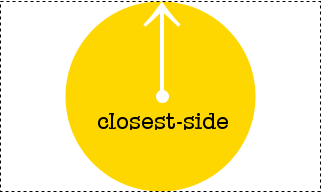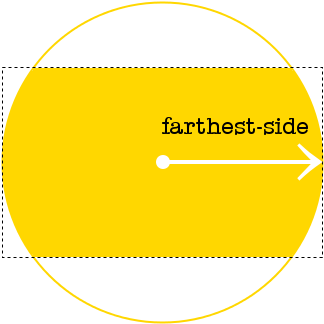# CSS circle() Function

The `circle()` function is a CSS basic shape value that's part of the CSS Shapes module.

Basic shapes such as `circle()` can be used as a value for properties such as `shape-outside` to control the flow of content around the element, and `clip-path` to clip the element's contents to the basic shape.

This means you can do things like, have text flowing around the element in the shape of a circle, have an image clipped to the shape of a circle, etc.

## Basic Example

Here's an example of how the `circle()` function works with the `shape-outside` property:

Here we have a `div` floated to the left. Now, because I've applied `shape-outside: circle()` to it, the subsequent text flows around the (invisible) circle that I specified.

It's easier to visualize this concept by looking at the following example.

As you can see, the circle is drawn within the floated box. The floated box actually protrudes out from the circle, into the surrounding text. But because we've applied `shape-outside: circle()`, the text is allowed to flow right up to the edges of the circle. The extent to which it does this depends on any argument that you pass to the `circle()` function.

This example doesn't use any arguments to specify the circle's radius. Therefore, it uses the default value (which is `closest-side`).

## How to Specify the Circle's Radius

You can specify the radius of the circle by passing an argument to the `circle()` function. As mentioned, `closest-side` is the default value. Here's how it works:The circle's radius will be based on the length from the center of the shape to the closest side of the reference box.

You can also specify `farthest-side`, which is calculated like this:In this case, the circle will base its radius on the length from the center of the shape to the farthest side of the reference box.

You can also use length values (such as `px`, `em`, `vw`, etc) and percentage values to specify the circle's radius.

Here's an example of using a percentage value.

## How to Position the Circle

You can specify a position for the circle by following the radius argument with a valid CSS position. This position specifies the circle's center. Just separate the radius and the position with `at`. Like this:

The center of this circle is 10 pixels from the left and 150 pixels from the top.

Here's a working example:

Position can be represented as a length value, a percentage value, or a combination of the `left`, `center`, `right`, `top`, and `bottom` keywords.

## Using `circle()` with `clip-path()`

Here's an example of using the CSS `circle()` function with the `clip-path` property.

If your browser supports the `clip-path` property (and the `circle()` function), the above image should appear in the shape of a circle. Otherwise it will appear as a normal rectangle shape.

At the time of writing, browser support for the `clip-path` property is limited. None of the major browsers support it, however, webkit browsers have partial support using the `-webkit-` prefix.

## Official Syntax

The official syntax of the `circle()` function is as follows:

And the syntax of `<shape-radius>` is:

The default value is `closest-side`.

The syntax for `<position>` is:

If only one value is specified, the second value is assumed to be `center`. If two values are given, a length or percentage as the first value represents the horizontal position (or offset) and a length or percentage as the second value represents the vertical position (or offset).

## Possible Values

The following values can be used to specify the circle's radius:

This can be specified in either of the following ways:
length
Specifies a length value to use (e.g. `px`, `em`, `vw`, etc) for the radius.
percentage
Specifies a percentage value to use. The percentage value uses the width and height of the reference box.
`closest-side`

This is the default value.

Specifies that the circle's radius will use the length from the center of the shape to the closest side of the box that uses the `circle()` function.

`farthest-side`

Specifies that the circle's radius will use the length from the center of the shape to the farthest side of the reference box.

position

The position argument defines the center of the circle. It is specified as a value from the top, left corner of the reference box using the values listed above (under Official Syntax).

## Browser Support

The following table provided by Caniuse.com shows the level of browser support for this feature.